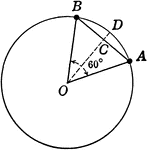### Circle With Sector and Segment labeled

Illustration of circle with sector and segment used to find area.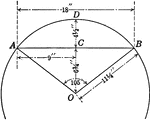### Circle With Sector and Segment labeled

Illustration of partial circle with sector and segment used to find area.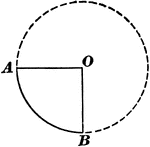### Sector of Circle

Illustration of a circle with a sector. "A sector of a circle is the space included between an arc and…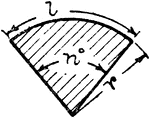### Sector Of Circle

Illustration of a sector of a circle. A sector is the space between an arc and two radii drawn to the…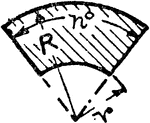### Sector Of A Hollow Circle

Illustration of a sector of a hollow circle. A sector is the space between an arc and two radii drawn…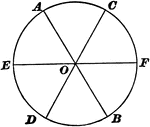### Sectors of Circle

Illustration of a circle with 6 equal sectors.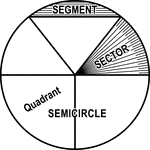### Circle with Segment, Semicircle, Sector, Quadrant, and Central Angle

Illustrations of a circle with segment, semicircle, sector, quadrant, and central angles drawn.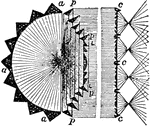### Condensing Light

"Fixed Conensing Light for a Single Sector, 1850.—The holophote Light pLp throws its whole light on…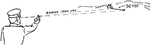### Finger system

"Used with indistinct or invisible targets and to define sectors." — Moss, 1914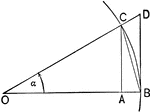### Triangles and Sectors in Quadrant I

Illustration of an angle &alpha with the vertex at the center, O, of a circle with radius OB. AC and…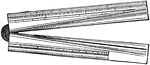### Sector

The sector is a hinged rule with scales used for various mathematical calculations.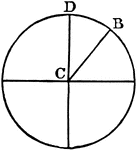### Sector

"In geometry: (a) A plane figure inclosed between the arc of a circle, ellipse, or other central curve…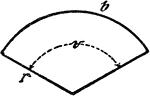### Circle Sector

An illustration showing a circle sector with radius r, center/central angle v, and length of circle…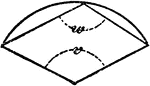### Circle Sector

An illustration showing a circle sector with center/central angle v and polygon angle w.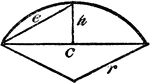### Circle Sector

An illustration showing a circle sector with height of segment h and radius r.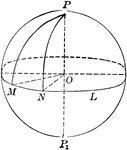### Sphere With Sectors and Arcs

Diagram of a sphere with sectors and arcs drawn.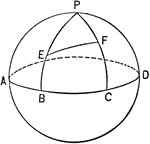### Sphere With Sectors and Arcs

Illustration of a sphere with sectors and arcs.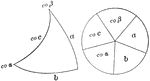### Napier's Right Spherical Triangle

Illustration of a right spherical triangle and the five circular parts placed in the sectors of a circle…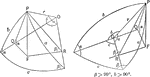### Relationships In A Spherical Triangle

Illustration used, with the law of sines, to find the relation between two sides of a spherical triangle…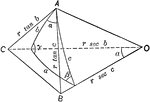### Relationships In A Spherical Triangle

Illustration used, with the law of cosines, to find the relation between the three sides and an angle…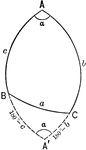### Relationships In A Spherical Triangle

Illustration used to extend the law of cosines when finding the relation between the three sides and…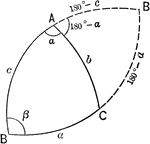### Relationships In A Spherical Triangle

Illustration used to extend the law of cosines when finding the relation between the three sides and…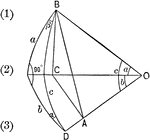### Right Spherical Triangle

Illustration of a right spherical triangle with a and b the sides, and α and β the angles…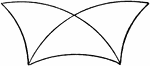### Superimposed Symmetrical Spherical Triangles

Diagram showing 2 superimposed symmetrical spherical triangles.### Symmetrical Spherical Triangles

Diagram showing 2 symmetrical spherical triangles.### Circle Inscribed In A Triangle

An illustration showing a circle with sectors D and E inscribed in a triangle with angles, A, B, and…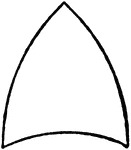### Spherical Triangle

Diagram showing a spherical triangle.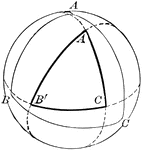### Spherical Triangle

Diagram showing a spherical triangle constructed by the intersection of polar arcs.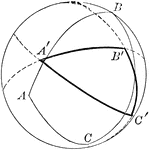### Spherical Triangle

Diagram showing a spherical triangle constructed by the intersection of polar arcs.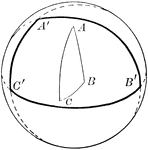### Spherical Triangle

Diagram showing a spherical triangle constructed by the intersection of polar arcs.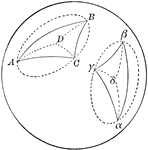### Two Symmetrical Spherical Triangles

Diagram showing a 2 symmetrical spherical triangles.